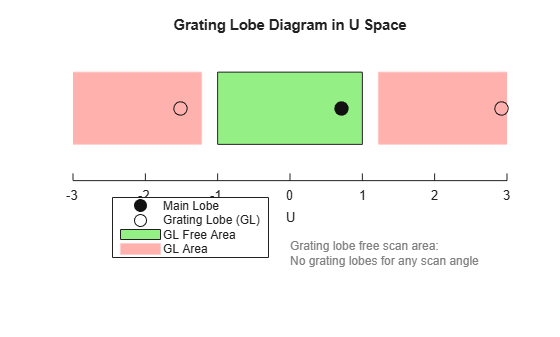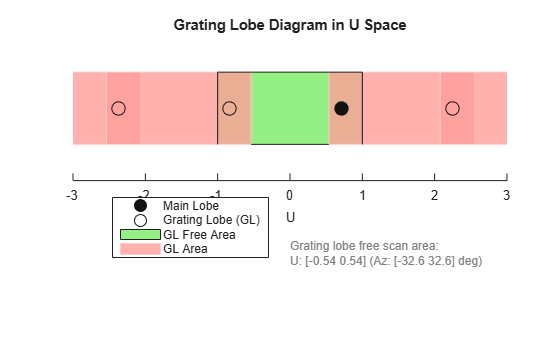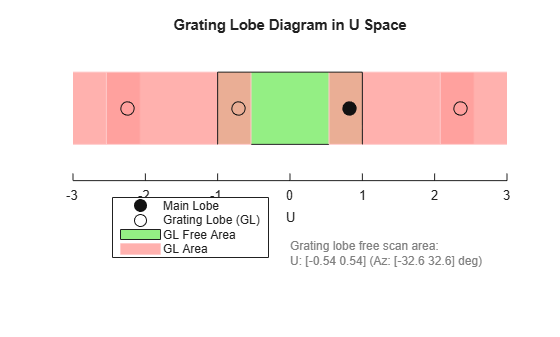# plotGratingLobeDiagram

System object: phased.ULA
Package: phased

Plot grating lobe diagram of array

## Syntax

```plotGratingLobeDiagram(H,FREQ) plotGratingLobeDiagram(H,FREQ,ANGLE) plotGratingLobeDiagram(H,FREQ,ANGLE,C) plotGratingLobeDiagram(H,FREQ,ANGLE,C,F0) hPlot = plotGratingLobeDiagram(___) ```

## Description

`plotGratingLobeDiagram(H,FREQ)` plots the grating lobe diagram of an array in the u-v coordinate system. The System object™ `H` specifies the array. The argument `FREQ` specifies the signal frequency and phase-shifter frequency. The array, by default, is steered to 0° azimuth and 0° elevation.

A grating lobe diagram displays the positions of the peaks of the narrowband array pattern. The array pattern depends only upon the geometry of the array and not upon the types of elements which make up the array. Visible and nonvisible grating lobes are displayed as open circles. Only grating lobe peaks near the location of the mainlobe are shown. The mainlobe itself is displayed as a filled circle.

`plotGratingLobeDiagram(H,FREQ,ANGLE)`, in addition, specifies the array steering angle, `ANGLE`.

`plotGratingLobeDiagram(H,FREQ,ANGLE,C)`, in addition, specifies the propagation speed by `C`.

`plotGratingLobeDiagram(H,FREQ,ANGLE,C,F0)`, in addition, specifies an array phase-shifter frequency, `F0`, that differs from the signal frequency, `FREQ`. This argument is useful when the signal no longer satisfies the narrowband assumption and, allows you to estimate the size of beam squint.

`hPlot = plotGratingLobeDiagram(___)` returns the handle to the plot for any of the input syntax forms.

## Input Arguments

 `H` Antenna or microphone array, specified as a System object. `FREQ` Signal frequency, specified as a scalar. Frequency units are hertz. Values must lie within a range specified by the frequency property of the array elements contained in `H.Element`. The frequency property is named `FrequencyRange` or `FrequencyVector`, depending on the element type. `ANGLE` Array steering angle, specified as either a 2-by-1 vector or a scalar. If `ANGLE` is a vector, it takes the form `[azimuth;elevation]`. The azimuth angle must lie in the range `[-180°,180°]`. The elevation angle must lie in the range `[-90°,90°]`. All angle values are specified in degrees. If the argument `ANGLE` is a scalar, it specifies only the azimuth angle where the corresponding elevation angle is 0°. Default: `[0;0]` `C` Signal propagation speed, specified as a scalar. Units are meters per second. Default: Speed of light in vacuum `F0` Phase-shifter frequency of the array, specified as a scalar. Frequency units are hertz When this argument is omitted, the phase-shifter frequency is assumed to be the signal frequency, `FREQ`. Default: `FREQ`

## Examples

expand all

Plot the grating lobe diagram for a 4-element uniform linear array having element spacing less than one-half wavelength. Grating lobes are plotted in u-v coordinates.

Assume the operating frequency of the array is 3 GHz and the spacing between elements is 0.45 of the wavelength. All elements are isotropic antenna elements. Steer the array in the direction 45 degrees in azimuth and 0 degrees in elevation.

```c = physconst('LightSpeed'); f = 3e9; lambda = c/f; sIso = phased.IsotropicAntennaElement; sULA = phased.ULA('Element',sIso,'NumElements',4,... 'ElementSpacing',0.45*lambda); plotGratingLobeDiagram(sULA,f,[45;0],c);```The main lobe of the array is indicated by a filled black circle. The grating lobes in the visible and nonvisible regions are indicated by empty black circles. The visible region is defined by the direction cosine limits between [-1,1] and is marked by the two vertical black lines. Because the array spacing is less than one-half wavelength, there are no grating lobes in the visible region of space. There are an infinite number of grating lobes in the nonvisible regions, but only those in the range [-3,3] are shown.

The grating-lobe free region, shown in green, is the range of directions of the main lobe for which there are no grating lobes in the visible region. In this case, it coincides with the visible region.

The white area of the diagram indicates a region where no grating lobes are possible.

Plot the grating lobe diagram for a 4-element uniform linear array having element spacing greater than one-half wavelength. Grating lobes are plotted in u-v coordinates.

Assume the operating frequency of the array is 3 GHz and the spacing between elements is 0.65 of a wavelength. All elements are isotropic antenna elements. Steer the array in the direction 45 degrees in azimuth and 0 degrees in elevation.

```c = physconst('LightSpeed'); f = 3e9; lambda = c/f; sIso = phased.IsotropicAntennaElement; sULA = phased.ULA('Element',sIso,'NumElements',4,'ElementSpacing',0.65*lambda); plotGratingLobeDiagram(sULA,f,[45;0],c);```The main lobe of the array is indicated by a filled black circle. The grating lobes in the visible and nonvisible regions are indicated by empty black circles. The visible region, marked by the two black vertical lines, corresponds to arrival angles between -90 and 90 degrees. The visible region is defined by the direction cosine limits $-1\le u\le 1$. Because the array spacing is greater than one-half wavelength, there is now a grating lobe in the visible region of space. There are an infinite number of grating lobes in the nonvisible regions, but only those for which $-3\le u\le 3$ are shown.

The grating-lobe free region, shown in green, is the range of directions of the main lobe for which there are no grating lobes in the visible region. In this case, it lies inside the visible region.

Plot the grating lobe diagram for a 4-element uniform linear array having element spacing greater than one-half wavelength. Apply a phase-shifter frequency that differs from the signal frequency. Grating lobes are plotted in u-v coordinates.

Assume the signal frequency is 3 GHz and the spacing between elements is 0.65 $\lambda$. All elements are isotropic antenna elements. The phase-shifter frequency is set to 3.5 GHz. Steer the array in the direction $4{5}^{\circ }$ azimuth, ${0}^{\circ }$ elevation.

```c = physconst('LightSpeed'); f = 3e9; f0 = 3.5e9; lambda = c/f; sIso = phased.IsotropicAntennaElement; sULA = phased.ULA('Element',sIso,'NumElements',4,... 'ElementSpacing',0.65*lambda ); plotGratingLobeDiagram(sULA,f,[45;0],c,f0);```As a result of adding the shifted frequency, the mainlobe shifts right towards larger $u$ values. The beam no longer points toward the actual source arrival angle.

The mainlobe of the array is indicated by a filled black circle. The grating lobes in the visible and nonvisible regions are indicated by empty black circles. The visible region, marked by the two black vertical lines, corresponds to arrival angles between ${\text{-}\text{90}}^{\circ }$ and ${\text{90}}^{\circ }$. The visible region is defined by the direction cosine limits $-1\le u\le 1$. Because the array spacing is greater than one-half wavelength, there is now a grating lobe in the visible region of space. There are an infinite number of grating lobes in the nonvisible regions, but only those for which $-3\le u\le 3$ are shown.

The grating-lobe free region, shown in green, is the range of directions of the main lobe for which there are no grating lobes in the visible region. In this case, it lies inside the visible region.

## Concepts

### Grating Lobes

Spatial undersampling of a wavefield by an array gives rise to visible grating lobes. If you think of the wavenumber, k, as analogous to angular frequency, then you must sample the signal at spatial intervals smaller than π/kmax (or λmin/2) in order to remove aliasing. The appearance of visible grating lobes is also known as spatial aliasing. The variable kmax is the largest wavenumber value present in the signal.

The directions of maximum spatial response of a ULA are determined by the peaks of the array’s array pattern (alternatively called the beam pattern or array factor). Peaks other than the mainlobe peak are called grating lobes. For a ULA, the array pattern depends only on the wavenumber component of the wavefield along the array axis (the y-direction for the `phased.ULA` System object). The wavenumber component is related to the look-direction of an arriving wavefield by ky = –2π sin φ/λ. The angle φ is the broadside angle—the angle that the look-direction makes with a plane perpendicular to the array. The look-direction points away from the array to the wavefield source.

The array pattern possesses an infinite number of periodically-spaced peaks that are equal in strength to the mainlobe peak. If you steer the array to the φ0 direction, the array pattern for a ULA has its mainlobe peak at the wavenumber value of ky0 = –2π sin φ0. The array pattern has strong grating lobe peaks at kym = ky0 + 2π m/d, for any integer value m. Expressed in terms of direction cosines, the grating lobes occur at um = u0 + mλ/d, where u0 = sin φ0. The direction cosine, u0, is the cosine of the angle that the look-direction makes with the y-axis and is equal to sin φ0 when expressed in terms of the look-direction.

In order to correspond to a physical look-direction, um must satisfy, –1 ≤ um ≤ 1. You can compute a physical look-direction angle φm from sin φm = um as long as –1 ≤ um ≤ 1. The spacing of grating lobes depends upon λ/d. When λ/d is small enough, multiple grating lobe peaks can correspond to physical look-directions.

The presence or absence of visible grating lobes for the ULA is summarized in this table.

Element SpacingGrating Lobes
λ/d ≥ 2No visible grating lobes for any mainlobe direction.
1 ≤ λ/d < 2Visible grating lobes can exist for some range of mainlobe directions.
λ/d < 1Visible grating lobes exist for every mainlobe direction.

 Van Trees, H.L. Optimum Array Processing. New York: Wiley-Interscience, 2002.